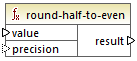# Altova MapForce 2021 Basic Edition

 round-half-to-even Scroll Home Prev Top Next More

The round-half-to-even function rounds the supplied number (first argument) to the degree of precision (number of decimal places) supplied in the optional second argument. For example, if the first argument is 2.141567 and the second argument is 3, then the first argument (the number) is rounded to three decimal places, so the result will be 2.141. If no precision (second argument) is supplied, the number is rounded to zero decimal places, that is, to an integer.

The "even" in the name of the function refers to the rounding to an even number when a digit in the supplied number is midway between two values. For example, round-half-to-even(3.475, 2) would return 3.48.## Languages

XQuery, XSLT 2.0.

## Parameters

Name

Type

Description

value

xs:decimal

Mandatory argument which provides the input value to be rounded.

precision

xs:integer

Optional argument which specifies the number of decimal places to round to. The default value is 0.